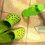# Anyone help me to solve this please

9 different books are to be arranged on a book-shelf. 4 of these books were written by Shakespeare, 2 by Dickens and 3 by Conrad. How many possible permutations are there if

(c) the books by Conrad are separated from each other?Note by Daniel Kua
4 years, 9 months ago

This discussion board is a place to discuss our Daily Challenges and the math and science related to those challenges. Explanations are more than just a solution — they should explain the steps and thinking strategies that you used to obtain the solution. Comments should further the discussion of math and science.

When posting on Brilliant:

• Use the emojis to react to an explanation, whether you're congratulating a job well done , or just really confused .
• Ask specific questions about the challenge or the steps in somebody's explanation. Well-posed questions can add a lot to the discussion, but posting "I don't understand!" doesn't help anyone.
• Try to contribute something new to the discussion, whether it is an extension, generalization or other idea related to the challenge.

MarkdownAppears as
*italics* or _italics_ italics
**bold** or __bold__ bold
- bulleted- list
• bulleted
• list
1. numbered2. list
1. numbered
2. list
Note: you must add a full line of space before and after lists for them to show up correctly
paragraph 1paragraph 2

paragraph 1

paragraph 2

[example link](https://brilliant.org)example link
> This is a quote
This is a quote
    # I indented these lines
# 4 spaces, and now they show
# up as a code block.

print "hello world"
# I indented these lines
# 4 spaces, and now they show
# up as a code block.

print "hello world"
MathAppears as
Remember to wrap math in $$ ... $$ or $ ... $ to ensure proper formatting.
2 \times 3 $2 \times 3$
2^{34} $2^{34}$
a_{i-1} $a_{i-1}$
\frac{2}{3} $\frac{2}{3}$
\sqrt{2} $\sqrt{2}$
\sum_{i=1}^3 $\sum_{i=1}^3$
\sin \theta $\sin \theta$
\boxed{123} $\boxed{123}$

Sort by:

Hi Daniel,

To answer your question, first of all we notice that order matters. The 6 Shakespeare and Dickens books (denoted by "O" below) can be put down in $6!=720$ ways. We then have 7 possible places to put the Conrad books down (on either side of the "O's"). Each Conrad book at a different X-spot however because the Conrad books need to be separated from each other.

X O X O X O X O X O X O X

As there are 7 possible places for the 1st Conrad book, 6 possible places for the 2nd Conrad book and 5 possible places for the 3rd Conrad book, the total number of permutations is $6! \times 7 \times 6 \times 5 = 151200$.

Kind regards,

Patrick Heebels

- 4 years, 8 months ago

This answer is true but i can't understand this method

- 2 months, 3 weeks ago

There are only 9 books it mean only 9 places then how can we put it into 7 plaves and then 6 places ?

- 2 months, 3 weeks ago

Sir i can't understand this question can you plz explain it?

- 2 months, 3 weeks ago

Hi Farah,

Suppose we have only 2 Shakespeare books and 1 Dickens book and we denote these as O1, O2 and O3. Then these books can be placed in 3! = 6 ways, namely:

O1-O2-O3 O1-O3-O2 O2-O1-O3 O2-O3-O1 O3-O1-O2 O3-O2-O1

Then suppose we have only 2 Conrad books that need to be separated from each other (these cannot be placed next to one another).

Fix one of the Shakespeare/Dickens permutations (denoted by "O" below). Then we have 4 places to place the first Conrad book and lets denote these places by the code K, L, M and N. We thus have:

K-O-L-O-M-O-N (The positions of the "O"s are occupied, the K/L/M/N are possibilities for the Conrad-books.)

The first Conrad book (C1) must be placed on the spot of K, L, M or N. Let's say this book (name it C1) is placed in position L. Then we have:

K-O-C1-O-M-O-N

The second Conrad book (C2) can now only be placed in the position of K, L or N. Thus there are 3 possibilities for the 2nd Conrad book.

All in all there are 3! x 4 x 3 = 72 permutations.

Now scale everything back up to 6 Shakespeare and Dickens books (resulting in the 6! factor). For the first Conrad book there are now 7 possibilities, which I denoted by an "X" in my original post. For each subsequent book there is one less option left.

Hopefully this clears up what you wanted to know. Let me know.

Kind regards, Patrick Heebels

- 2 months, 3 weeks ago

May be....plz let me know the ans

- 4 years, 9 months ago

210

- 4 years, 9 months ago